This page has been robot translated, sorry for typos if any. Original content here.

# The concept of alternating current

Foreword

Before you start talking about meters, remember that these are electrical appliances, and in the following description I will rush into all sorts of smart terms. Of course, if you have an electrical education, then this part can be skipped right away. And if you only know about electricity, if you plug the iron into a power outlet it starts to heat up, then I highly recommend reading this chapter so that you don’t feel like a stranger at a universal holiday of life. The chapter is taken from the site Voropaev E.G. "Electrical Engineering" , there are many more, I recommend reading for self-education, it is written quite popularly (at the level of the first year of the electrical technical school).

So:

Definition: Variables are called currents and voltages that change in time, in magnitude and direction. Their value at any time is called the instantaneous value. Instantaneous values ​​are indicated in small letters: i, u, e, p.

Currents whose values ​​are repeated at regular intervals of time are called periodic. The smallest period of time after which their repetitions are observed is called the period and is denoted by the letter T. The inverse of the period is called the frequency, i.e.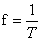and measured in hertz (Hz). Value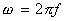called the angular frequency of the alternating current, it shows the phase change of the current per unit time and is measured in radians divided by second

The maximum value of alternating current or voltage is called amplitude. It is denoted in capital letters with the index `` m '' (for example, I m ). There is also the concept of the rms value of alternating current (I). Quantitatively, it is equal to: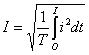It should be noted that the actual voltage value is less than the maximum.

Alternating current can be mathematically written as: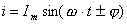Here, the index expresses the initial phase. If a sinusoid starts at the intersection of the coordinate axes, then = 0, then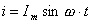The initial current value can be to the left or right of the ordinate axis. Then the initial phase will be leading or lagging.

#### 1.2. RESISTANCE IN AC CHAINS.

Electric current in conductors is continuously connected with magnetic and electric fields.
Elements that characterize the conversion of electromagnetic energy into heat are called active resistances (denoted by R).
Elements associated with the presence of only a magnetic field are called inductances.
Elements associated with the presence of an electric field are called capacities.
Typical representatives of active resistances are resistors, incandescent lamps, electric furnaces, etc.
Inductance is possessed by relay coils, windings of electric motors and transformers. Inductance is calculated by the formula: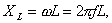where L is the inductance.
Capacitors, long power lines, etc. possess capacity.
Capacitance is calculated by the formula: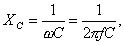where C is the capacity.
Real consumers of electric energy can have a complex value of resistances. In the presence of R and L, the value of the total resistance Z is calculated by the formula: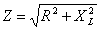Similarly, Z is calculated for the chain R and C: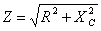Consumers with R, L, C have a total resistance of: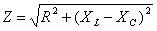#### 1.3. SERIAL CONNECTION OF ACTIVE RESISTANCE R, CAPACITOR C AND INDUCTION L

Consider a circuit with active, inductive and capacitive resistances connected in series (Fig. 1.3.1).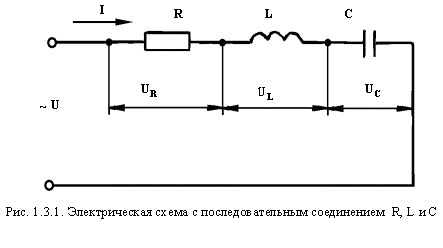To analyze the circuit, we decompose the network voltage U into three components:
U R - voltage drop across the active resistance,
U L is the voltage drop across the inductive resistance,
U C is the voltage drop across the capacitance.

The current in circuit I will be common to all elements: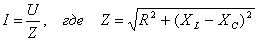Verification is carried out according to the formula: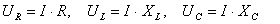It should be noted that voltages in individual parts of the circuit do not always coincide in phase with current I.
So, at the active resistance, the voltage drop coincides in phase with the current, at the inductive phase it is 90 ° ahead of the phase and at the capacitive one it is 90 ° behind it.
Graphically, this can be shown in the vector diagram (Fig. 1.3.2).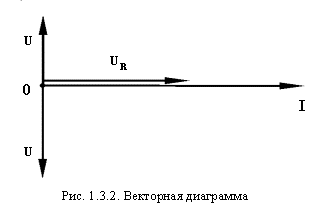The three stress drop vectors depicted above can be geometrically combined into one (Fig. 1.3.3).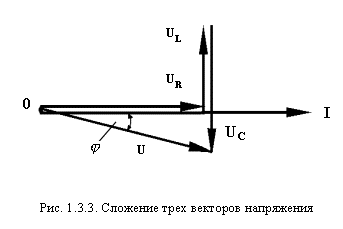In such a combination of elements, active-inductive or active-capacitive characters of the circuit load are possible. Consequently, the phase shift has both a positive and a negative sign.
An interesting mode is when = 0.
In this case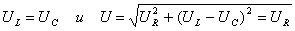This mode of operation of the circuit is called voltage resonance.
The impedance at voltage resonance has a minimum value: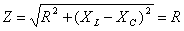, and at a given voltage U, current I can reach a maximum value.
From the condition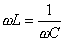define the resonant frequency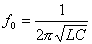Stress resonance phenomena are widely used in radio engineering and in individual industrial installations.

#### 1.4. PARALLEL CONNECTION OF CONDENSER AND COIL, Possessing active resistance and inductance

Consider a parallel circuit of a capacitor and a coil with active resistance and inductance (Fig. 1.4.1).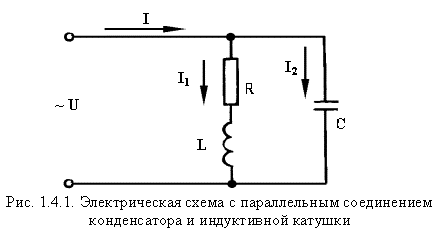In this circuit, the common parameter for the two branches is the voltage U. The first branch, the inductive coil, has an active resistance R and an inductance L. The resulting resistance Z 1 and current I 1 are determined by the formula: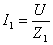where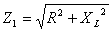Since the resistance of this branch is complex, the current in the branch lags in phase from the voltage by an angle.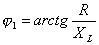We show this in the vector diagram (Fig. 1.4.2).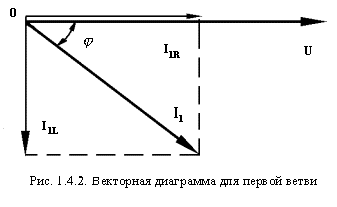We project the current vector I 1 on the coordinate axis. The horizontal component of the current will be the active component I 1R , and the vertical - I 1L . Quantitative values ​​of these components will be equal to: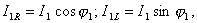Where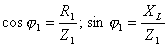A capacitor is included in the second branch. His resistance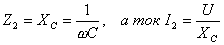This current is 90 ° ahead of phase in voltage.
To determine the current I in the unbranched part of the circuit, we use the formula: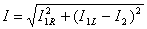Its value can also be obtained graphically by adding the vectors I 1 and I 2 (Fig. 1.4.3)
The shear angle between current and voltage is denoted by the letter j .
Here, various modes of operation of the circuit are possible. At = + 90 °, the capacitive current will prevail, at = -90 ° - inductive.
Possible mode when = 0, i.e. the current in the unbranched part of circuit I will be active. This will happen in the case when I 1L = I 2 , i.e. with equal reactive components of the current in the branches.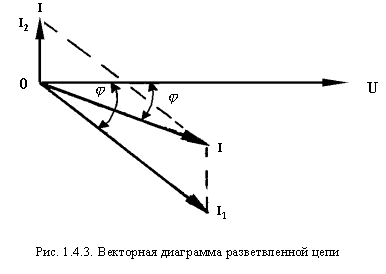On the vector diagram it will look like this (Fig. 1.4.4):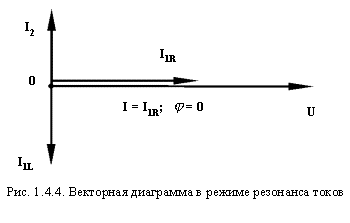This mode is called current resonance. As in the case of a resonance of stresses, it is widely used in radio engineering.
The case of parallel connection of R, L and C considered above can also be analyzed from the point of view of increasing cos j for electrical installations. It is known that cos j is a technical and economic parameter in the operation of electrical installations. It is determined by the formula: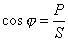where

P is the active power of electrical installations, kW,
S - full power of electrical installations, kW.
In practice, cos j is determined by taking active and reactive energy readings from the counters, and dividing one reading into another gives tg j .
Further, according to the tables, cos j is also found .
The more cos j , the more economical the power system works, since at the same current and voltage values ​​(for which the generator is designed), it can get more active power from it.
The decrease in cos j leads to incomplete use of equipment and at the same time the efficiency of the installation decreases. Electricity tariffs provide a lower cost of 1 kilowatt-hour at high cos j , compared to low.
Cos enhancement activities include:
- prevention of idling electrical equipment,
- full load of electric motors, transformers, etc.
In addition, cos j has a positive effect on the connection of static capacitors to the network.